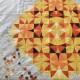# Cathedral Window Patchwork Maths – How Many Windows?

The number of ‘window panes’ in a traditional cathedral window quilt is not the same as the number of background squares. It is many, many more! So how do you know how many fabric squares to cut? Here is the Arithmetic!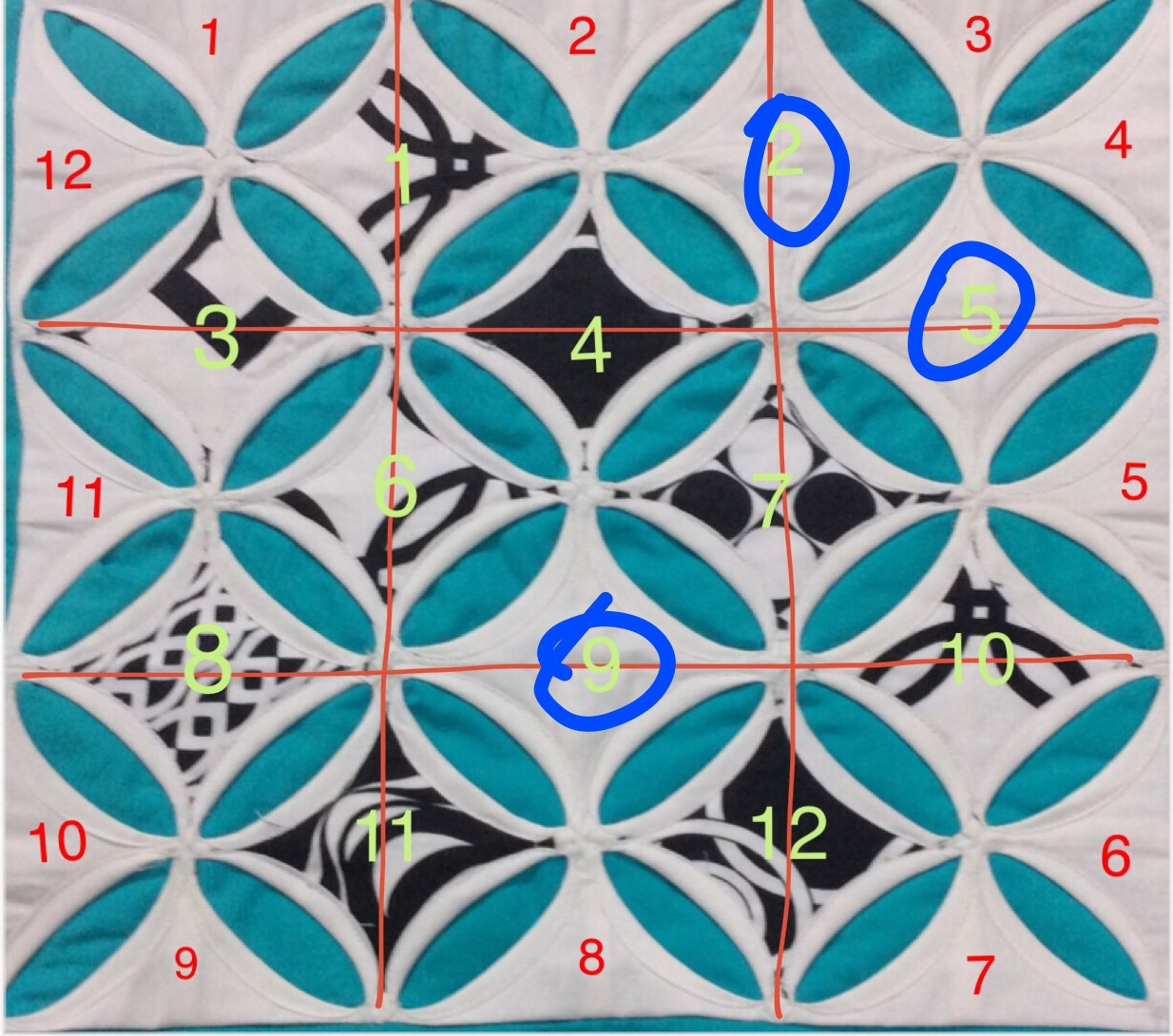Caution: Loaded with primary school level Arithmetic. Read at your peril ( or if  you plan to make a traditional cathedral window quilt).

There are plenty of tutorials which tell you how to make a traditional window quilt, including mine, here. There are also tutorials which tell you, given the finished square design, how big your background fabric square and window (pane) square should be.  For example, if you want to finish with a 5″ square, your background fabric should be 10.5″ square, and the window fabric 3″. This is the tutorial I referred to for measurements for my current project.
However, did you know that the number of window squares you need are not equal to the number of background squares in your quilt? What was that again? Well I was working with these 3×3 patchwork pieces, and I cut 9 window patches for each. The result is here for you to see!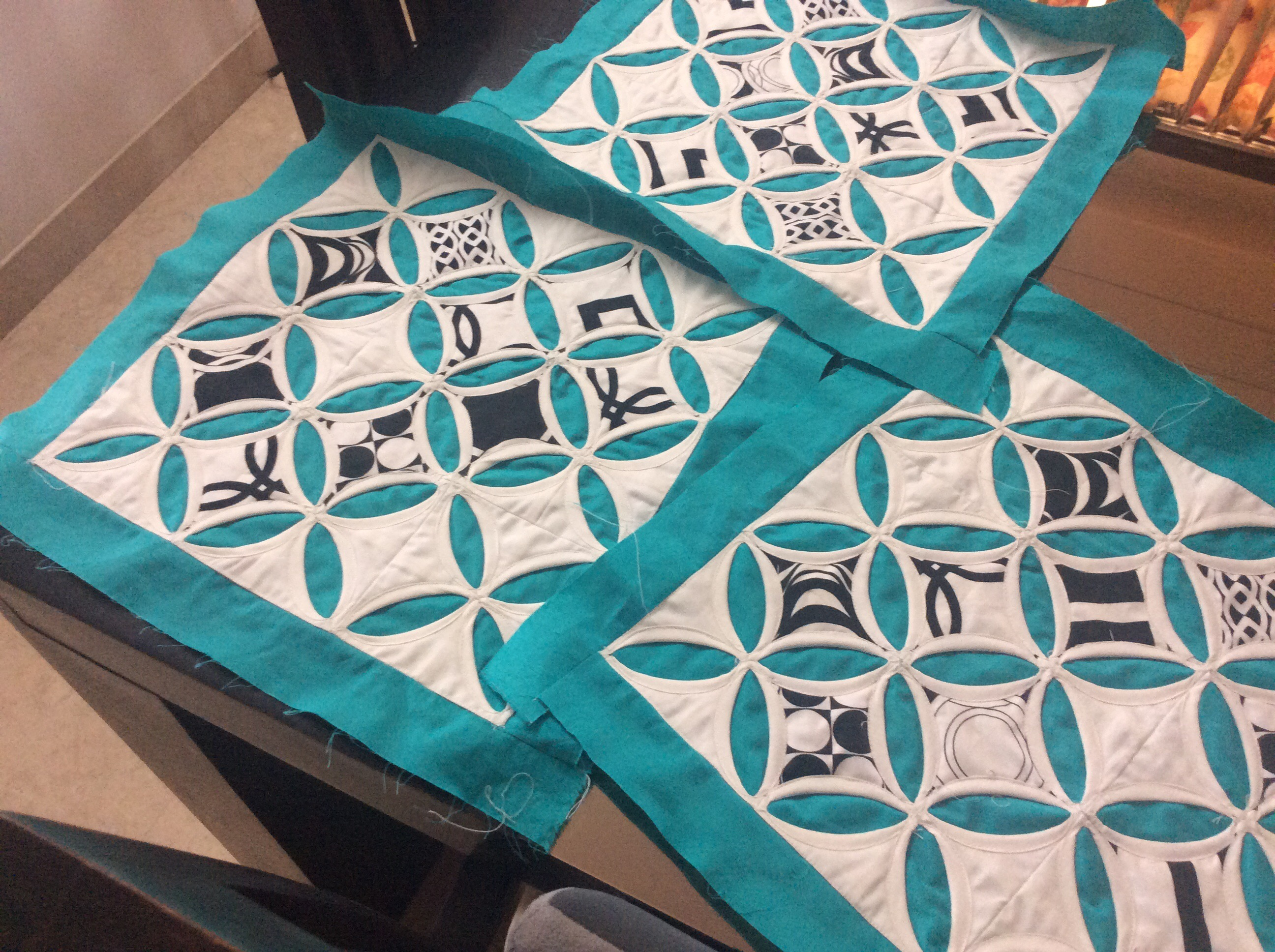There are three blank ( white) window ‘panes’ in each. I should have cut 12 window (black and white) squares for the centre windows. If I wanted the printed fabric in the (half) windows on the edges, I would have had to cut 12 more squares ( which I would have folded diagonally and attached to the ‘frames’).

Things get even more complicated when you are working on a larger quilt.  If you are working with, say, 24 squares and making a 6×4 or 2×12 or 3×8 quilt, you would now have guessed that you need more than just 24 window squares.  But, did you know that the number of windows is different for each one of these? And none of them is 24.

So how does one do the Math? If you are one of those people who just can’t wrap their head around figures, well…I guess there is nothing to be done but to cut the fabric in batches as you go along. It is impossible for me to make a chart here with all the possible block configurations!

For the others, here is the way it works!

The Arithmetic

All you need to know is

– the total number of background squares you are working with and

– the configuration of your quilt. What is meant by configuration?  If the total number of squares is 36, you could be joining them in a 6×6  or 4×9 or 3×12 configuration.

It does not matter what the size of your squares is.

Step 1. Multiply the total number of squares in your quilt by 4. If my quilt has 36 squares, I will get the figure of 144.

Step 2. Calculate the number of ‘half’ window panes at the edges. This is equal to the total number of squares at the edges (perimeter).

If my quilt configuration is 6 x6, the total number of half window panes is  6 + 6 + 6 + 6 = 24

If my quilt configuration is 4×9 , the total number of half window panes is 4 + 9 + 4 + 9 =  26

If my quilt configuration is 3×12, the total number of half window panes is 3 + 12 + 3 + 12 = 30

If I plan to add a ‘pane’ fabric to these half window panes, I need to cut these many squares in addition to the rest I will be cutting. ( Like mentioned earlier, these will be folded diagonally and attached to the window, with the diagonal fold at the edge. )

Step 3. Subtract the number of half window panes from the figure obtained at Step #1. This result is divided by 2 to get the number of full window panes for the centre!

If my quilt configuration is 6×6, the total number of half window panes is 24. If I subtract this from 144 , I get 120, which I divide by 2 to get 60! So that is the number of window fabric squares I need!

For the 4×9 configuration, I get 144 minus 26, which is 118 and the number squares will be 59.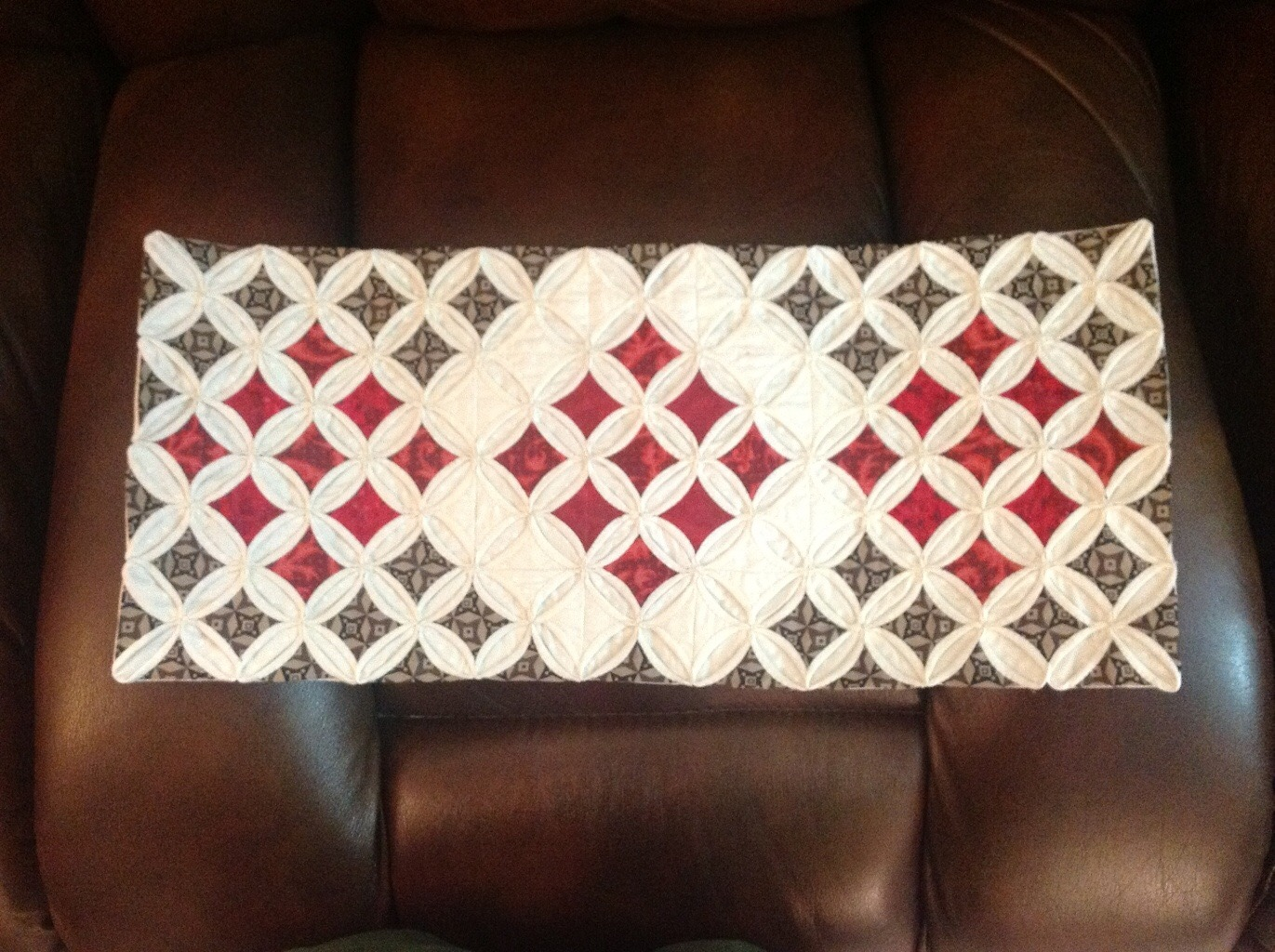The 4 by 9 configuration. Ignoring the 26 brown half squares at the edges, can you count 59 window squares in the centre, including the 12 left blank?

For the 3×12 configuration, it will be 57 squares in the centre and 30 ( half) squares at the edges. Check this out!

Not so complicated, right? Let us crosscheck this with my 3×3 cushion cover!

Cross-checking the Calculations
Here is my cushion cover, with the nine blocks in a 3×3 configuration.

Had I added the black and white printed fabric to the half windows at the edges,  I would have needed 12 squares ( folded diagonally into half) for those.The number of panes at the edge are equal to the number of blocks along each of the edges totaled together.

As for the centre squares, I subtract 12 from 36 ( total number of background squares multiplied by 4) to get 24 and halve it to get 12!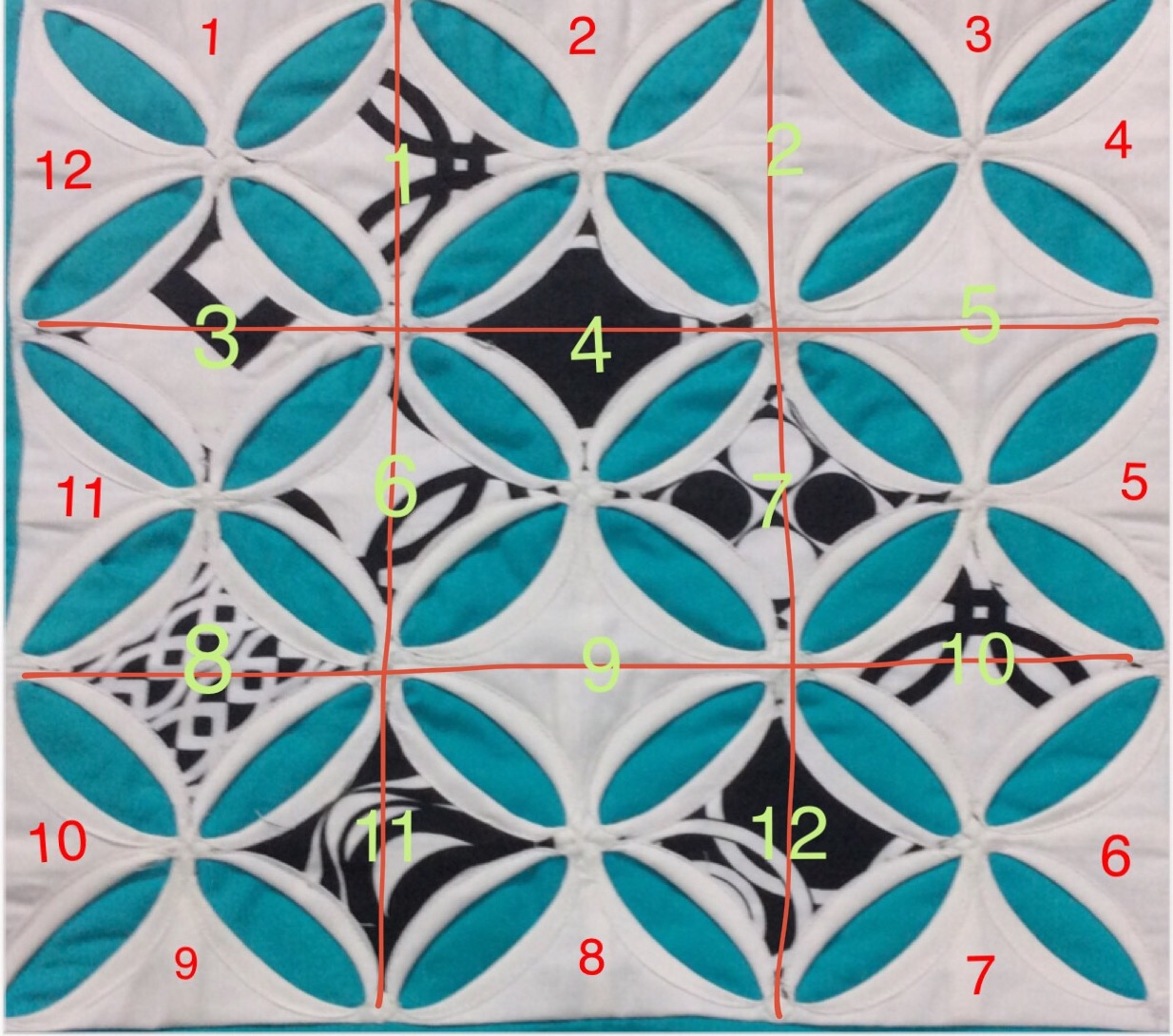That is 12 printed fabric window squares needed for the centre panes ( marked in lime green).

But I had cut only 9 black & white printed squares.

Explains my 3 missing squares quite neatly, doesn’t it?